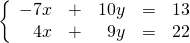Chapter 5: Systems of Equations

# 5.3 Addition and Subtraction Solutions

One of the most powerful methods for solving systems of equations (finding their intersection points) is in adding and subtracting equations. In later math courses, this process is the foundation of matrix algebra, but for now, consider only equations.

The objective in finding the solutions to the these systems of equations is to isolate variables and find what they are equal to. Adding and subtracting equations can make this process quite fast and easy.

Example 5.3.1

Find the solution to the following system of equations: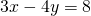and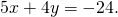First, line them up over top of each other, since they will be added or subtracted. Notice that, when added, the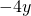and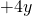cancel each other out: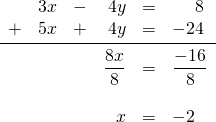It is now known that these equations intersect at the value where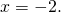Now choose one of the two original equations (generally, the simplest to work with) and substitute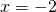to find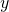: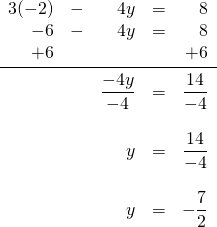The intersection point of these two linear equations isand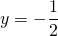, or at the coordinate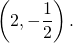Generally, it takes a little more work than just placing the equations on top of each other and having a variable cancel out. In most cases, there is typically one variable that, once multiplied, is cancelled out when the equations are added to each other. For instance:

Example 5.3.2

Find the solution to the following system of equations: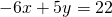and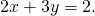First, line up the equations and choose the variable that shall be eliminated: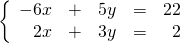The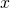variable could be eliminated if the bottom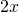were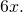For this to happen, the entire bottom equation would have to be multiplied by 3: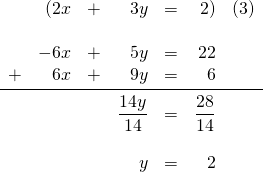It is now known that these equations intersect at the value where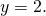Now choose one of the two original equations (the simplest looks to be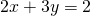) and substitute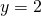to find: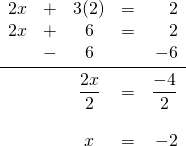The intersection point of these two linear equations isand, or at the coordinate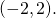The more difficult of systems of two linear equations generally require the manipulation of both equations to eliminate one of the variables. For example, consider the following pair of linear equations:

Example 5.3.3

Find the solution to the following system of equations: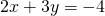and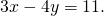First, line up the equations and choose the variable that shall be eliminated: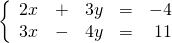It looks the simplest to eliminate thevariable. This means the top equation needs to be multiplied by 3 and the bottom equation multiplied by −2. Then, the two equations are added together, and each side is divided by 17: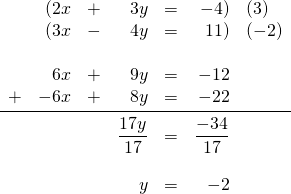It is now known that these equations intersect at the value where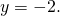Now choose one of the two original equations (choose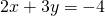) and substitute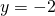to find: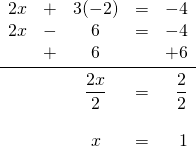The intersection point of these two linear equations is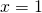and, or at the coordinate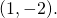The last examples that will be done for this topic are equations having no solution or infinite solutions.

Example 5.3.4

Find the solution to the following system of equations: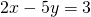and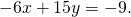First, line up the equations to choose the variable that shall be eliminated: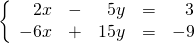To eliminate thevariable, the top equation needs to be multiplied by 3: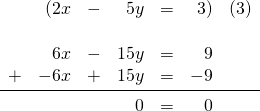Everything cancels out because the two equations are identical. Therefore, there are infinite solutions.

Example 5.3.5

Find the solution to the following system of equations: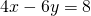and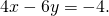Once these two equations are aligned, it is easy to see they are identical except for their intercepts. They are parallel lines. To cancel the variables out, one of the two equations must be multiplied by −1: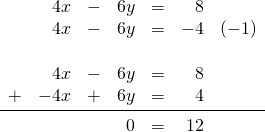The result is all the variables cancelling out to 0 and falsely equalling some number. There is no solution, since these equations will never intercept each other.

# Questions

For questions 1 to 24, solve each system of equations by elimination.

1.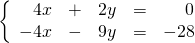2.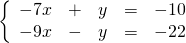3.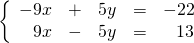4.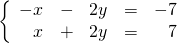5.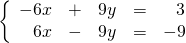6.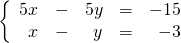7.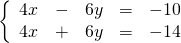8.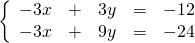9.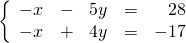10.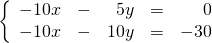11.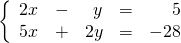12.13.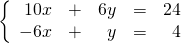14.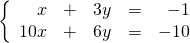15.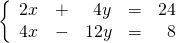16.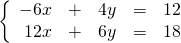17.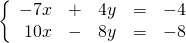18.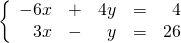19.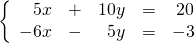20.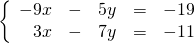21.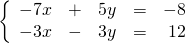22.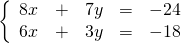23.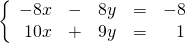24.